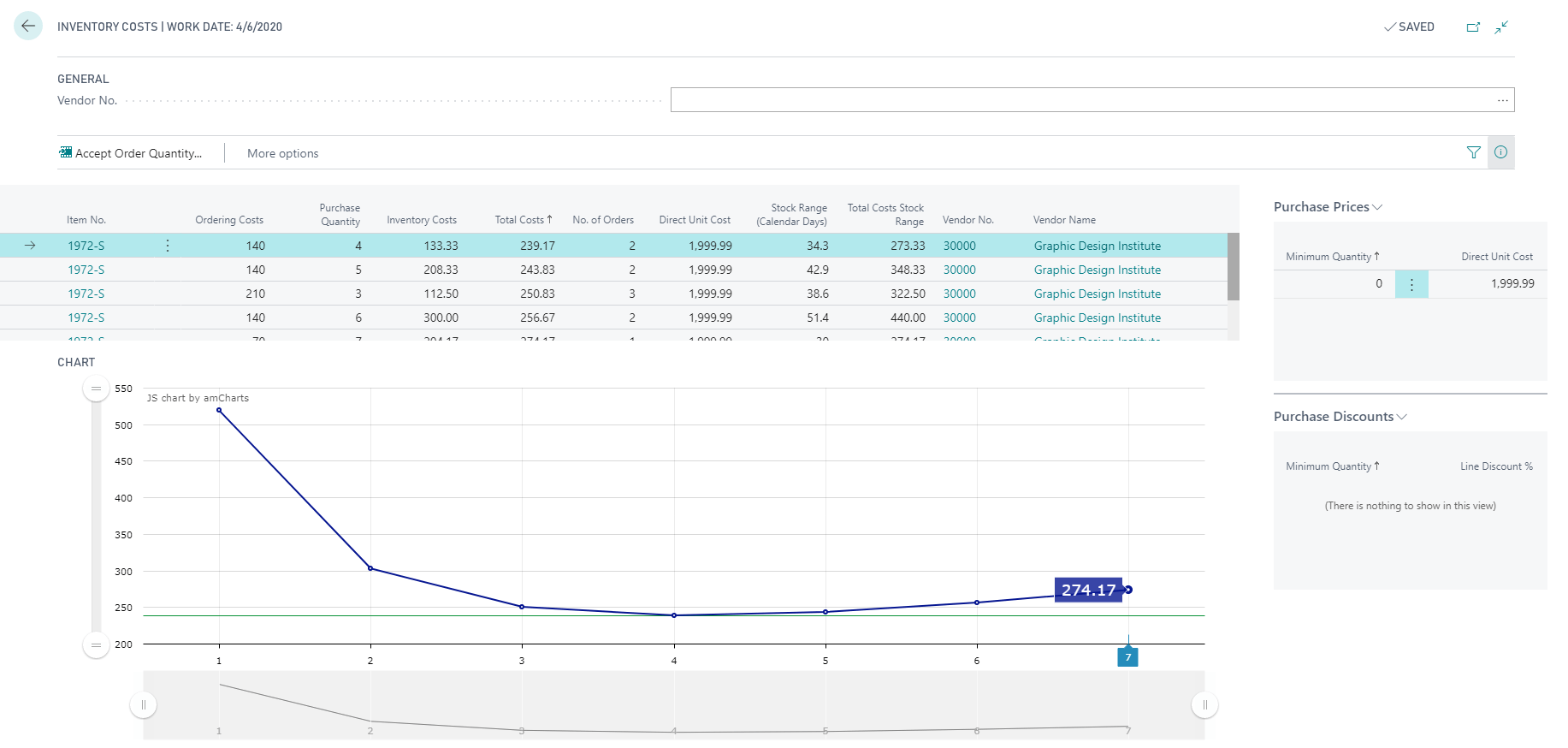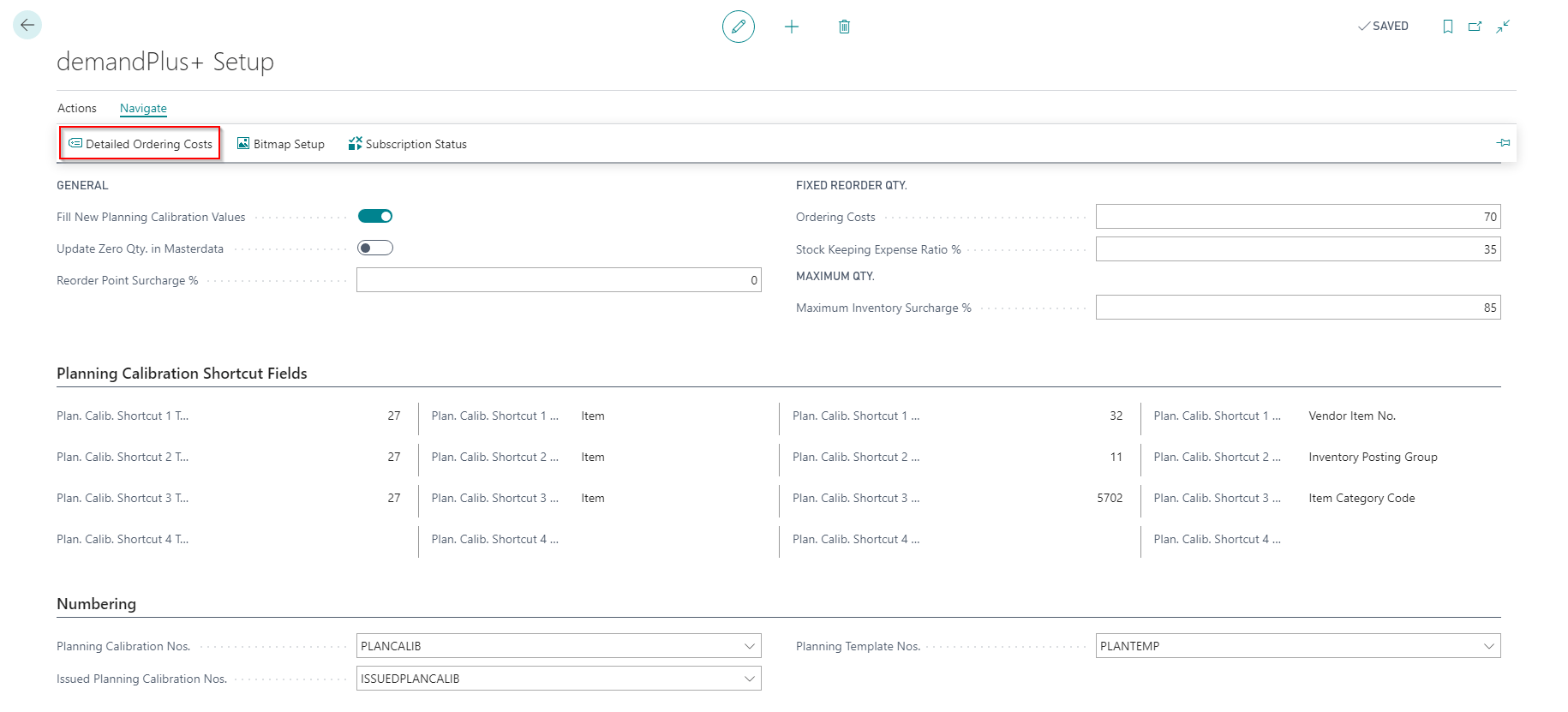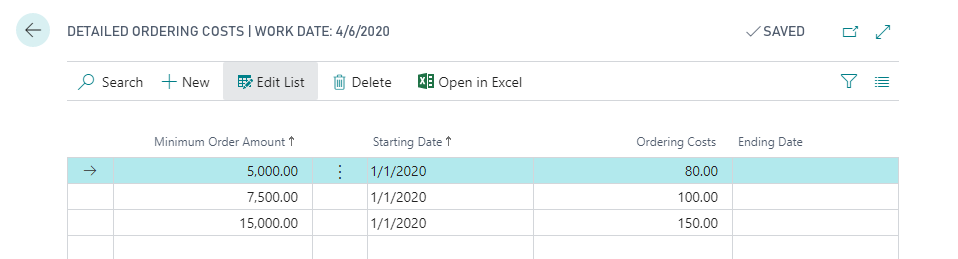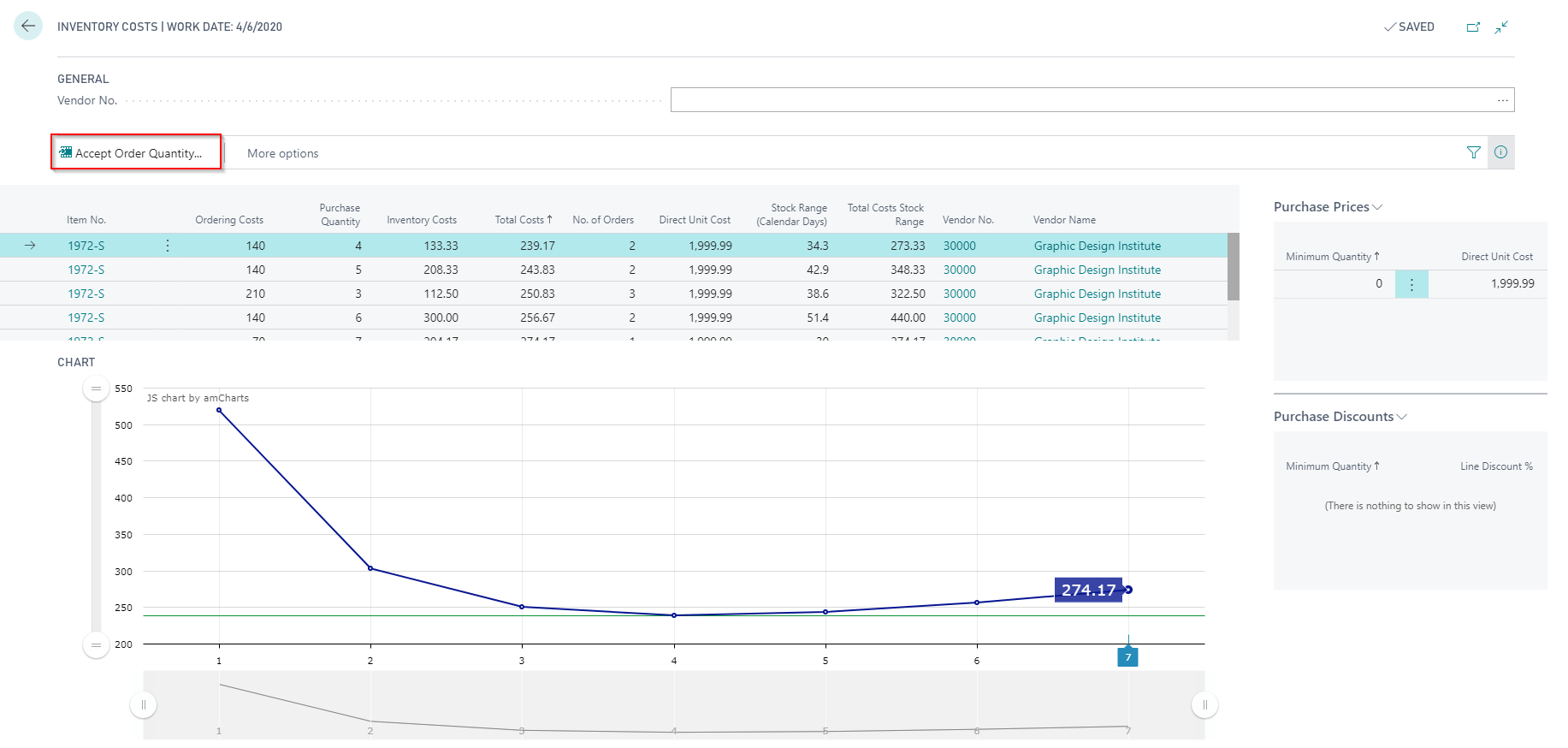# Inventory Costs

### Content

Detailed Ordering Costs

Inventory Costs

Chart

Factboxes

The inventory costs are calculated for each period of the planning calibration. They are the basis for calculating the reorder quantities. By clicking on action “Inventory costs”Note: You will find the same action in the period lines. This allows you to view the inventory costs per period.

### Detailed Ordering Costs

You have the possibility to set up detailed ordering costs. To do so, go to the demandPlus+ Setup and choose “Detailed Ordering Costs”:You can store detailed ordering costs for a time interval and a minimum order amount. These values are taken into account when calculating the inventory costs.

###Inventory Costs

The costs are sorted in descending order of total costs. All combinations are calculated for all item vendors. The optimal order quantity is the quantity that causes the lowest total costs. (Disregarding the vendor)

 Name Description Calculation Item No. Indicates the number of the item, according to the defined number series. Variant Code Specifies the variant of the item. Ordering Costs Processing costs of the orders. No. of orders * Ordering costs from the setup table. Purchase Quantity Calculated quantity for this order combination. All possible combinations (Order Multiple) are taken into account so that the requirements for this period are covered. Total Costs Indicates the total cost of the order combination, based on the number of calendar days. Total cost = (A / B) * C A = Total Costs Stock Range B = Stock Range (Calendar Days) C = Calendar Days No. of Orders Number of orders that will be created in the planning period Direct Unit Cost Specifies the direct unit cost for this order quantity. Stock Range (Calendar Days) Indicates the stock range in calendar days. (No. of Orders * Purchase Quantity) / Average Usage per Calendar Day Stock Range (Workdays) Indicates the stock range in working days. (No. of Orders * Purchase Quantity) / Average Usage per Working Day Inventory Costs Total inventory costs of the ordering quantity in the warehouse. Inventory Costs = (A * B * C) / 360 A = Direct Unit Cost * Purchase Quantity/ 2  => Ø-Stock value B = Stock Keeping Expense Ratio from demandPlus+ setup / 100% C = Stock Range (Calendar Days) Note: “Safety Stock Qty.” is ignored because it is not relevant for the calculation of Order Quantity. Total Costs Stock Range Indicates the sum of order costs and inventory costs. Order Costs + Inventory Costs Vendor No. Specifies the code of the vendor who delivers this item by default. Vendor Name Specifies the name of the vendor who delivers this item by default.

If you want to transfer a different Order Quantity to the Planning Calibration, you can use the action “Accept Order Quantity”.### ChartThe chart visualizes Total Costs. X-axis = “Order Quantity”, Y-axis = “Total Costs”.
In addition, the green line shows the calculated optimum.
All Inventory Costs are displayed. If you want to filter the chart in relation to a vendor, you can use the filter field in the header section.

### Factboxes

In the fact boxes, the prices and discounts used for calculation are displayed.